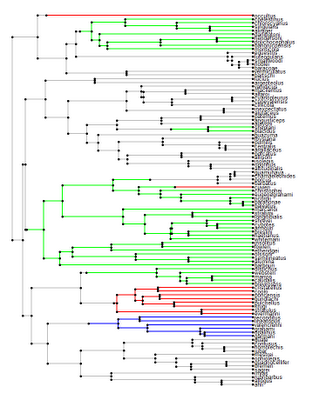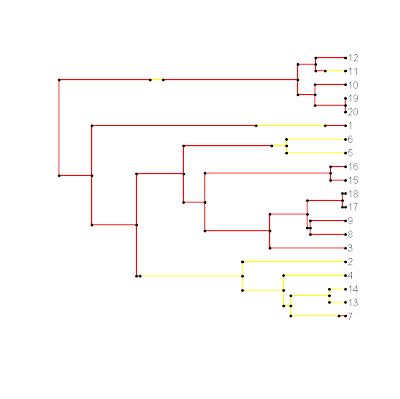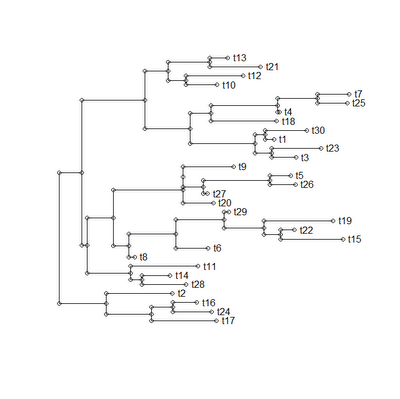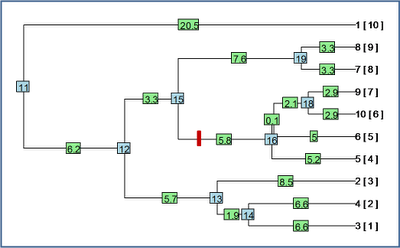## Wednesday, June 29, 2011

### Using detach() to unload a library

Say we have a current session of R open, and, lo and behold, there is a new version of "phytools" available. If we download the binaries and try to install, e.g.:

> install.packages("phytools_0.0-3.zip",repos=NULL)

we get the following error:

Warning: package 'phytools' is in use and will not be installed

This can be avoided simply by unloading the library before installing, and the reloading the new version afterwards. This is accomplished as follows:

> detach(package:phytools)
> install.packages("phytools_0.0-3.zip",repos=NULL)
package 'phytools' successfully unpacked and MD5 sums checked
> require(phytools)

Of course, this also applies to packages installed from a CRAN mirror.

## Tuesday, June 28, 2011

### Slicing a tree to create all subtrees

Someone recently emailed me about slicing a tree at a particular height from the root and then keeping all the subtrees. This seemed pretty easy, so I thought I would implement it using the {ape} function extract.clade(). This is how I did it. First, I reordered the tree "cladewise", using reorder.phylo(); next, I computed the heights of all the internal and terminal nodes in the tree and put these values into a matrix to match the \$edge matrix from my "phylo". Then, I found all the edges intersected by my slice and identified the tipward nodes on those edges, excluding all the nodes that were also tips. Finally, I extracted each subtree descended from the nodes using extract.clade() and added a \$root.edge to each tree depending on where its root branch was sliced. I then returned all of these trees as a "multiPhylo" object.

The function is posted to my website, here. Please be warned that I'm not sure what will happen if the slice point is greater than the tree length or if it only intersects terminal edges.

First, let's create a random tree:

> require(geiger)
> tree<-birthdeath.tree(b=1,d=0,taxa.stop=20)Now, let's slice it at slice=0.6, shown as the dotted line above (having verified, of course, that this will indeed produce some non-trivial subtrees):

> source("treeSlice.R")
> trees<-treeSlice(tree,0.6)
> plot(trees,root.edge=T)

which, in this case, produces the following set of three trees:We're done!

### plotSimmap() & make.simmap() added to new version of "phytools"

I just posted a new version (0.0-3) of the "phytools" package (still in "alpha" release from my website only). This version contains a couple of minor updates as well as the addition of make.simmap() (to generate stochastic character mapped trees) and plotSimmap() (to plot them), both of which I hope prove to be handy functions.

### Anolis biogeography stochastically mapped on the Caribbean phylogeny

I thought I would give both make.simmap() and plotSimmap() a practical go, so I decided to map & plot a plausible scenario for island colonization in the Caribbean - shown below:I made a couple of updates to both make.simmap() and plotSimmap() to make this possible.

First, in the ML transition matrix, estimated inside make.simmap() using ace(), some of the transition rates were 0.0 in the full, symmetric model for these data. This makes sense, given the large number of states (four - for the four main islands in the Greater Antilles) and small number of transitions between states. I decided to allow additional models - in this case, using the equal-rates model:

> mtree<-make.simmap(tree,x,model="ER")

Next, plotting a relatively large tree created some graphical problems. First, I eliminated all the whitespace around the plot by setting the graphical parameter, par()\$mar within the function:

par(mar=c(0.1,0.1,0.1,0.1))

Finally, I added the option to manipulate the font size for the tip labels. This just changes the graphical parameter cex.

> plotSimmap(mtree,fsize=0.7)

for instance.

I will shortly post these updated versions of make.simmap() and plotSimmap() to my R phylogenetics page and I will add them to the next version of "phytools."

### Plot stochastic character mapped tree

I just completed a beta version of my plotSimmap() function. I'll post a little more about how this was accomplished tomorrow, but it was fairly straightforward after figuring out how to plot rooted phylograms generally (see previous post here). To check it out, download it (source here), load the source into R, and then run the following code (for example):

> require(geiger)
> require(phytools)
> tree<-drop.tip(birthdeath.tree(b=1,d=0,taxa.stop=21),"21")
> x<-sim.char(tree,model.matrix=list(matrix(c(-1,1,1,-1),2,2)),
model="discrete")[,,1]
> mtree<-make.simmap(tree,x)
> legend<-c("red","yellow"); names(legend)<-c(1,2)
> source("plotSimmap.R")
> plotSimmap(mtree,legend)## Monday, June 27, 2011

### Plotting rooted trees

Now that I have a function to generate stochastic character mapped trees, I thought I would try and see if there would be an easy way to plot them - that is without having to first transform the tree object into another format. [For instance, if I printed the tree to file, I could then read it into FigTree, which I believe can plot mutationally mapped trees; similarly, Rich Fitzjohn tells me that {diversitree} has SIMMAP plotting capabilities.]

To venture down this road, I first had to try to figure out how trees are plotted. I'll be the first to admit that this is largely untrodden territory for me (with the possible exception of my phylomorphospace plotting function). This was this morning's adventure, and I have just posted a function, plotTree(), which (if not pretty) seems to do the trick.

A few comments on the process. First, getting the lengths of edges, as well as their horizontal positions, is easy. In fact, I had already solved this in an earlier function. Second, the tricky part was figuring out vertical spacing and ordering. Some clues about how to do this are helpfully provided in Felsenstein's (2004) book chapter on plotting trees. This proved to be invaluable in the end.

The way we do this is first by reordering the tree "cladewise" (using reorder.phylo() from the {ape} package) and then spacing the tips of the tree out evenly. The height of all the terminal edges is now assigned. Next, we reorder the tree "pruningwise" - this allows us to now go from the tips of the tree to the root, encountering each internal node before its predecessor. Each time, we assign a vertical position for that node (and the corresponding preceding edge) as the average position of the two descendants, in a binary tree (or the average of the first and the last in a tree with multifurcations). This is the "intermediate" algorithm given by Felsenstein (2004; p. 574) Finally, we connect all the horizontal edges with vertical lines, and add tip labels if inclined.

Even though this plotting function just does more or less the same thing (but slower) as {ape}'s plot.phylo(), I have posted the code here for interested readers. Example execution code and plot are given below:

> require(ape)
> source("plotTree.R")
> tree<-rtree(25)
> plotTree(tree)## Friday, June 24, 2011

### New version of phytools; PDF manual

I just added the function make.simmap() and the latest version of brownie.lite() to {phytools} and posted the updated version online here. I also fixed a bunch of errors in the help files. In addition, I have posted the phytools PDF manual (which basically just compiles the DESCRIPTION file and all the help pages) here. Please feel free to post feedback or comments.

### Using make.simmap() with brownie.lite()

Now that make.simmap() seems to work, I thought I would give a quick demo of how it can be used in conjunction with fastBM() and brownie.lite(), as well as a couple of functions from the {geiger} package, to simulate and then estimate under O'Meara et al.'s (2006) multi-rate Brownian motion model.

First, we load the dependencies (and source):
> require(phytools)
> require(geiger)
> source("make.simmap.R")

Now, let's simulate a tree and our discrete character:
> tree<-drop.tip(birthdeath.tree(b=1,d=0,taxa.stop=101),"101")
> x<-sim.char(tree,model.matrix=list(matrix(c(-0.2,0.2,0.2,-0.2) ,2,2)),model="discrete")[,,1]

We can create a stochastic character mapped tree for x using make.simmap():
> mtree<-make.simmap(tree,x)

Now, we take advantage of the way this map is stored to simulate on the tree. We'll use a rate of 1.0 for branches in state "1", and rate 10.0 for branches in state "2".
> simtree<-tree
> simtree\$edge.length<-mtree\$mapped.edge[,"1"]+ mtree\$mapped.edge[,"2"]*10
> y<-fastBM(simtree)

Finally, we fit the model using brownie.lite():
> result<-brownie.lite(mtree,y)
> result
\$sig2.single
 3.410848
\$a.single
...
\$logL1
 -186.3361
\$k1
 2
\$sig2.multiple
1 2
0.953248 8.705116
...
\$logL.multiple
 -161.2660
\$k2
 3
\$P.chisq
 1.431489e-12
\$convergence
 "Optimization has converged."

Pretty cool!

P.S., I will shortly post a version of brownie.lite(), that uses box-constraints on the optimization via optim(). This seems to be more stable. It also returns ancestral values for the root node of the tree under each model.

## Thursday, June 23, 2011

A couple of things to add to the last post:

1) I have "confirmed" that \$lik.anc in ace() from the {ape} package does indeed return the (rescaled) conditional likelihoods in the sense given on p. 254 of Felsenstein's (2004) book. When I say "confirmed," I do so with quotes - because I mean I worked a simple numerical example by hand (using the so-called "peeling algorithm" of Felsenstein (1981)), and the values I obtained (once rescaled to sum to 1.0) were numerically identical to those in \$lik.anc. This is good, I think, because these are the values we need for our mapping algorithm.

2) Next, I wanted to add that the tree object produced by make.simmap() is in the modified "phylo" format that I developed for read.simmap(). This is described in more detail here. The format can still be read by {ape}'s functions (which will ignore the additional elements we have added to the object), but it can also be used by brownie.lite() and evol.vcv(). So far I have ignored writing and plotting these stochastic character mapped trees, but I will work on this soon.

3) Finally, make.simmap() relies on the "cladewise" edge order, and will probably not work properly if the edges are in "pruningwise" order. For more information about these orders see the help page for reorder.phylo() in {ape}. "cladewise" is the order produced by read.tree(), but "cladewise" order is created by many functions in the package {phangorn}. To reorder a tree in "pruningwise" order, simply type:

> tree<-reorder.phylo(tree)

## Wednesday, June 22, 2011

### Stochastic character mapping on the tree

I'm just now returning from the 'Evolution' meeting (joint meeting of SSE, ASN, and SSB) in Norman, Oklahoma. I saw many good and exciting talks at the meeting. Hopefully I will be able to channel some of this excitement into new functions and methods for my nascent {phytools} package in the coming weeks.

Rich Fitzjohn, a Ph.D student with Sally Otto at UBC, gave an excellent talk on fitting and using state-dependent diversification models; however, he also began his talk with a very clear and simple explanation of the algorithm for stochastic character mapping, originally developed by Nielsen (2002) and Huelsenbeck et al. (2003). A couple of my functions (in particular, brownie.lite(), which implements the method of O'Meara et al. (2006); and evol.vcv(), which implements Revell & Collar (2009)) take stochastic map trees created by my function read.simmap() as input.

As it turns out, Rich has already programmed stochastic character mapping in his impressive package {diversitree} that I am just beginning to explore; however after Rich's explanation the exercise seemed to be so straightforward that I thought I would try it myself. This way it will be easy for users to seamless go from discrete and continuous character vectors to the rate variation analyses of brownie.lite() and evol.vcv().

To conduct stochastic character mapping we first need the conditional likelihoods of each of our states for our mapped discrete character at each node in the tree. Making the task even easier, it is my understanding that these are returned as the object \$lik.anc by the {ape} function ace(...,type="discrete"). [Note, this is not well documented - so I am still trying to confirm that these likelihoods are indeed the "right" ones.] Once we have these, we just traverse the tree once, from the root to the tips. At the root, we just assign the root state with probability determined by the relative conditional likelihoods at each root. Then, for each node, we compute the element-wise product of the vector of conditional likelihoods for that node and the vector Pi·, where P for a branch length t is given by: eQ*t; and then we stochastically assign internal nodes using the relative probabilities we have computed. (Here, eX denotes the matrix exponential of X.)

After we have obtained a set of probable states for all the nodes of the tree, we then generate plausible character histories along each edge. We do this by generating waiting times for character shifts from an exponential distribution with rate -Qii for starting state i. We do this along each edge of the tree, rejecting branches that don't start and finish with the states simulated in the previous step of our analysis.

I have just posted a beta version of this function to my R phylogenetics page (direct link here). Assuming that there doesn't prove to be any major bugs in the function, I will also incorporate into the next version of {phytools}.

To use the function, simply load the source, and then type:

> mtree<-make.simmap(tree,x)

for "phylo" object tree and discrete character vector x then cross your fingers.* [*This is not a required step, but it can never hurt.] Right now the function fits only a general time-reversible model (i.e., symmetric Q, all rates different); but in future I will add additional options.

## Wednesday, June 15, 2011

### New version of phytools package available, now with documentation files!!

I just posted a new "alpha" version of my phytools package to my R-phylogenetics page - now with documentation (i.e., "man") pages. In addition to still (hopefully) being able to install the package from source (either using my directions, here, or by following the helpful suggestions of my readers); Windows users can also now install the package binaries as follows. First, download phytools_0.0-1.zip; then type:

> install.packages("phytools_0.0-1.zip",repos=NULL)

Writing even the sparse documentation that I created for the present version of the package was a lot of work (Paradis' extensive documentation for {ape} now seems even more impressive), so I look forward to getting feedback.

## Monday, June 13, 2011

### Re-rooting along a terminal edge

Ok, my wrapper function, reroot(), also now can re-root the tree along terminal edges. The way I did this was by re-rooting the tree at the parent node of the target edge; dropping the target tip; then creating a new two taxon "phylo" object with the target edge split between an edge leading to the target tip and the new root edge for the remainder of the tree. Finally, I used bind.tree() to reattach the remaining tree to the two taxon "phylo" object.

The code is simple enough, so I have a reduced version (without error handling, etc.) below. I will post a completed version shortly to my R-phylogenetics page.

Code below:

reroot<-function(tree,node.number,position){
if(node.number>length(tree\$tip)){
# first, re-root the tree at node.number
tr<-root(tree,node=node.number,resolve.root=T)
# now re-allocate branch length to the two edges descending
# from the new root node
b<-sum(tr\$edge.length[tr\$edge==(length(tree\$tip)+1)])
tr\$edge.length[tr\$edge==(length(tree\$tip)+1)]<-c(position, b-position)
} else {
# first, root the tree at the parent of node.number
tr1<-root(tree,node=tree\$edge[match(node.number, tree\$edge[,2]),1])
# now drop tip
tr1<-drop.tip(tr1,tree\$tip.label[node.number])
# create phylo object
tr2<-list(edge=matrix(c(3L,1L,3L,2L),2,2,byrow=T), tip.label=c(tree\$tip.label[node.number],"NA"), edge.length=c(tree\$edge.length[match(node.number,tree\$edge[,2])] -position,position),Nnode=1)
class(tr2)<-"phylo"
tr<-bind.tree(tr2,tr1,where=which(tr2\$tip.label=="NA"))
}
return(tr)
}

Let's try it out. First, a random birth-death tree:Here, the blue numbers are internal node numbers; the green numbers are edge lengths; and the numbers in [ square parentheses ] after each tip label are the node numbers for the tips.

First, let's try and re-root this tree 1.0 units of edge length along the branch leading to node #16 (marked with a red hashmark on the figure above):

> test1<-reroot(tree,node.number=16,position=1.0)
> plot(test1)Cool - it seems to work.

Now, let's try re-rooting the tree along a terminal edge - say the edge leading to taxon "2". We'll do this 8.0 units along this edge (which is ~8.5 units long in total). We can actually do this without knowing the node number for taxon "2" as follows:

> test2<-reroot(tree,node.number=which(tree\$tip.label=="2"), position=8.0)
> plot(test2)And we're done.

I just wrote this simple function this morning, but it is for a project I am presently working on, so if you find any bugs - I would be happy to hear about it.

### Re-rooting a tree along an edge

This morning I'm trying to figure out how to re-root a tree along an edge - more specifically how to re-root a phylogeny at a specific position along an edge. To re-root a tree at an internal node (and thus with a basal polytomy) we can use the very handy {ape} function root(). Furthermore, root(...,resolve.root=TRUE) will root a tree along any internal edge, but it assigns the full branch length to only one of the descendant branches from the new root. Rooting along an internal edge of the tree is thus the easiest problem to solve. The following very simple wrapper function for root() seems to do the trick:

reroot<-function(tree,node.number,position){
# first, re-root the tree at node.number
tr<-root(tree,node=node.number,resolve.root=T)
# now re-allocate branch length to the two edges descending
# from the new root node
b<-sum(tr\$edge.length[tr\$edge==(length(tree\$tip)+1)])
tr\$edge.length[tr\$edge==(length(tree\$tip)+1)]<-c(position,b-position)
return(tr)
}

Try it out!

The next problem is figuring out how to re-root the tree along a terminal edge. This is going to be a little trickier, but I think I will be able to do this using root(), drop.tip(), and bind.tree() (although suggestions are, of course, welcome).

## Tuesday, June 7, 2011

### R phylogenetics workshop

Luke Harmon & Mike Alfaro just posted an announcement of their intensive short course on R for phylogenetic comparative methods (details here). My impression is that this course is intended for graduate students and postdocs, so I thought it might be of interest to some readers of this blog. Luke Harmon & I go way back, and I can attest that, along with doing great work in method development, Luke is also a fantastic instructor. Please contact him for more information.

## Wednesday, June 1, 2011

### "Alpha" version of phytools package now available

I just wanted to let you know that I have just posted the first "alpha" version of my "phytools" (phylogenetic tools for comparative biology - and other things) package online at my R phylogenetics page (direct link to zipped tarball here).

To install, simply download the file, navigate to the appropriate directory, and then type:

> install.packages("phytools_0.0-1.tar.gz",type="source")

The package can then be loaded as normal, i.e.,

> require(phytools)

or

> library(phytools)

in the current or future R sessions.

Please let me know if you find any difficulties with functions loaded this way, rather than downloaded and loaded from their individual source files. (Or, of course, any difficulties in general.) I look forward to comments! (Even a report of successful installation is more than welcome.)

Unfortunately, I have not yet written man pages for these functions, so this will have to come in the first "beta" version release of "phytools" - hopefully some time soon.